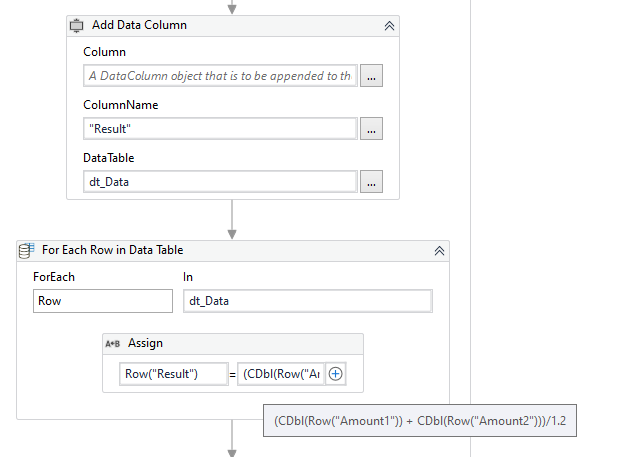# How to execute/perform array formula (Ctrl+Shift+Enter) also known as CSE Formula in the most optimal way?

Greetings, currently the design of the process is I print the formulas in a DT and write it into the excel file where the formula will be referring its value to. This works perfectly with basic formulas such as “=(A1+B1)/1.2” then I print the DT in Cell C1 but recently I discovered that some complex formulas requires to be an Array formula (CSE Formula) which is performed by pressing hotkey combinations “Ctrl”+“Shift”+“Enter” after inputting the formula instead of pressing “Enter” which the outcome will enclose the entered formula in curly brackets, eg. “{=(A1+B1)/1.2}”. I addressed this with my team which is the approach was to use Type Into activity to type in the formula, then Send Hotkey Activity to perform the “Ctrl”+“Shift”+“Enter”, my issue with this approach is that I print the formula to thousands of rows eg. 15000 rows, which may be slow. Are there any other optimal way for this?

Regards,
Wil

We can work on our data table variable only and then write the output in an excel file.Refer the xaml

CSEFormula.xaml (7.6 KB)